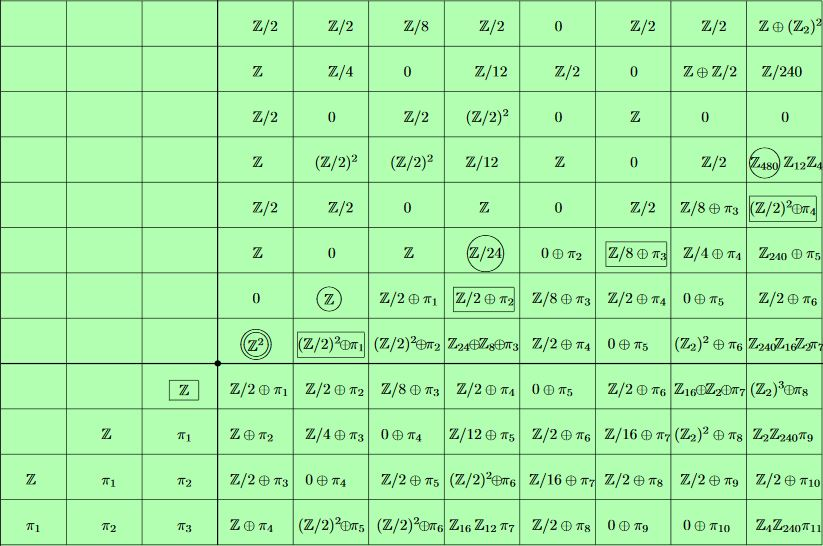# nLab equivariant sphere spectrum

Contents

### Context

#### Stable Homotopy theory

stable homotopy theory

Introduction

# Contents

#### Representation theory

representation theory

geometric representation theory

# Contents

## Idea

Its RO(G)-graded homotopy groups are the equivariant version of the stable homotopy groups of spheres.

## Definition

The $G$-equivariant sphere spectrum is the equivariant suspension spectrum of the 0-sphere $S^0 = \ast_+$

$\mathbb{S} = \Sigma^\infty_G S^0$

for $S^0$ regarded as equipped with the (necessarily) trivial $G$-action. It follows that for $V$ an orthogonal linear $G$-representation then in RO(G)-degree $V$ the equivariant sphere spectrum is the corresponding representation sphere $\mathbb{S}(V) \simeq S^V$.

(e.g. Schwede 15, example 2.10)

## Properties

### Equivariant homotopy groups

Just as for the plain sphere spectrum, the equivariant homotopy groups of the equivariant sphere spectrum in ordinary integer degrees $n$ are all torsion, except at $n = 0$:

$\pi_n^H(\mathbb{S})\otimes \mathbb{Q} = \left\{ \array{ \cdots & for \; n = 0 \\ 0 & otherwise } \right.$

But in some RO(G)-degrees there may appear further non-torsion groups, see the examples below.

In degree 0, the tom Dieck splitting applied to the equivariant suspension spectrum $\mathbb{S} = \Sigma^\infty_G S^0$ gives that $\pi_0^G(\mathbb{S})$ is the free abelian group on the set of conjugacy classes of subgroups of $G$:

(1)$\pi_0^G(\mathbb{S}) \simeq \underset{[H \subset G]}{\oplus} \pi_0^{W_G H}(\Sigma_+^\infty E (W_G H)) \simeq \mathbb{Z}[conjugacy\;classes\;of\;subgroups]$

(e.g. Schwede 15, p. 64)

## Examples

### $\mathbb{Z}_2$-equivariance

Consider $G= \mathbb{Z}_2$ the cyclic group of order 2 and write $\pi^S_{p,q}$ for the homotopy group in RO(G)-degree given by the representation on $\mathbb{R}^{p+q}$ with $\mathbb{Z}_2$ acts by reflection on the first $p$ coordinates, and trivially on the remaining $q$ coordinates:

The following groups contain $\mathbb{Z}$-summands:

In summary and more generally we have the following $\mathbb{Z}/2$-equivariant stable homotopy groups of spheres in low bidegree:The table shows the $\mathbb{Z}/2$-equivariant stable homotopy groups of spheres $\pi^S_{p,q}$ with $p+q$ increasing horizontally to the right, and $p$ increasing vertically upwards. The origin is the double-circled $\pi^S_{0,0} = \mathbb{Z}^2$. The complex Hopf fibration $\hat\eta$ generates $\pi^S_{1,0} = \mathbb{Z}$, and the quaternionic Hopf fibration generates $\pi^S_{2,1} = \mathbb{Z}/24$

graphics grabbed from Dugger 08, based on Araki-Iriye 82

(beware that Dugger 08 uses a different bi-degree labeling convention: the $(p,q)$ here is $(p+q,p)$ in Dugger 08, matching the coordinates of the above table)

$n_{sgn} + n$$0$$1$$2$$3$
$n_{sgn}$$\pi^{st}_{n_{sgn} + n}$
$2$$0$$0$$\mathbb{Z} = \langle (h_{\mathbb{C}})^2\rangle$$\mathbb{Z}_{24} = \langle h_{\mathbb{H}}\rangle$
$1$$0$$\mathbb{Z} = \langle h_{\mathbb{C}}\rangle$$\pi_1^{st} \oplus \mathbb{Z}_2$$\pi^{st}_2 \oplus \mathbb{Z}_2$
$0$$\pi_0^{st} \oplus \mathbb{Z}$$\pi_1^{st} \oplus (\mathbb{Z}_2)^2$$\pi_2^{st} \oplus (\mathbb{Z}_2)^2$$\pi_3^{st} \oplus \mathbb{Z}_8 \oplus \mathbb{Z}_{24}$

### Cyclic ($G_D \hookrightarrow SO(2)$-)equivariance

The global equivariant sphere spectrum for all the cyclic groups over the circle group is canonically a cyclotomic spectrum and as such is the tensor unit in the monoidal (infinity,1)-category of cyclotomic spectra (see there).

### General

General lecture notes include

Discussion in rational equivariant stable homotopy theory includes

The sphere spectrum in global equivariant homotopy theory is discussed in

### $\mathbb{Z}/2$-equivariance

Discussion of $G$-equivariant homotopy groups for $G = \mathbb{Z}/2$ is in

• Peter Landweber, On Equivariant Maps Between Spheres with Involutions, Annals of Mathematics Second Series, Vol. 89, No. 1 (Jan., 1969), pp. 125-137 (jstor)

• Shôrô Araki, Kouyemon Iriye, Equivariant stable homotopy groups of spheres with involutions. I, Osaka J. Math. Volume 19, Number 1 (1982), 1-55. (Euclid:1200774828)

• Kouyemon Iriye, Equivariant stable homotopy groups of spheres with involutions. II, Osaka J. Math. Volume 19, Number 4 (1982), 733-743 (euclid:1200775536)

• Daniel Dugger, Daniel Isaksen, $\mathbb{Z}/2$-equivariant and R-motivic stable stems, Proceedings of the American Mathematical Society 145.8 (2017): 3617-3627 (arXiv:1603.09305)

with exposition in

### $\mathbb{Z}/4$-equivariance

Discussion for $G = \mathbb{Z}/4$ is in

• M. C. Crabb, Periodicty in $\mathbb{Z}/4$ equivariant stable homotopy theory, in Mark Mahowald and Stewart Priddy (eds.) Algebraic Topology, Proceedings of the International Conference held March 21-24 (1988) Contemporary Mathematics, volume 96, 1989 (pdf, publisher page)

General background includes